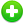#Canada Inflation Calculator

Rapidly calculate how the buying power of Canadian dollar has changed over time from year 1914 to present.

Year Dollar
In
In

If in 2010 You purchased an item for \$200 CAD then in 2021 that same item would cost \$247.9 CAD## How to Calculate the Canada Inflation Rate

Calculates the equivalent value of the Canada dollar (CAD) in any year from 1914 to present. Calculations are based on average CPI. This inflation calculator uses the following formula to calcualte the Canada inflation rate.

Inflation Rate = ( CPI2 - CPI1 )CPI1 × 100

Where,

CPI - consumer price index.

CPI2 - The consumer price index in the second period.

CPI1 - The consumer price index in the previous period.

This Calculator uses monthly consumer price index data from 1914 to the present to show changes in the cost of a fixed basket of consumer purchases.

Canada Consumer Price Index (CPI) Table